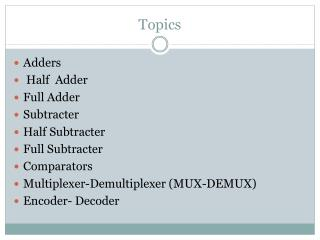Download PresentationTopics

# Topics

Download Presentation## Topics

- - - - - - - - - - - - - - - - - - - - - - - - - - - E N D - - - - - - - - - - - - - - - - - - - - - - - - - - -
##### Presentation Transcript

1. Topics Adders Half Adder Full Adder Subtracter Half Subtracter Full Subtracter Comparators Multiplexer-Demultiplexer (MUX-DEMUX) Encoder- Decoder

2. Introduction Arithmetic circuits are the ones which perform arithmetic operations like addition, subtraction, multiplication, division, parity calculation. Most of the time, designing these circuits is the same as designing muxers, encoders and decoders.    In the next few pages we will see few of these circuits in detail.

4. Half Adder Adding two single-bit binary values X, Y produces a sum S bit and a carry out C-out bit. This operation is called half addition and the circuit to realize it is called a half adder. Truth Table SUM=X(XOR)Y ; CARRY=X(AND )Y

5. Symbol

6. Circuit

7. Full Adder Full adder takes a three-bits input. Adding two single-bit binary values X, Y with a carry input bit C-in produces a sum bit S and a carry out C-out bit.

8. K-map K-map for SUM K-MAP FOR CARRY CARRY = XY + XZ + YZ SUM = X'Y'Z + XY'Z' + X'YZ‘

9. Full Adder using AND-OR Gates Circuit for Carry CARRY = XY + XZ + YZ Circuit for SUM SUM = X'Y'Z + XY'Z' + X'YZ‘

11. Subtracter Subtracter circuits take two binary numbers as input and subtract one binary number input from the other binary number input. Similar to adders, it gives out two outputs, difference and borrow (carry-in the case of Adder). There are two types of subtracters. Half Subtracter. Full Subtracter.

12. Half Subtracter The half- subtracter is a combinational circuit which is used to perform subtraction of two bits. It has two inputs, X (minuend) and Y (subtrahend) and two outputs D (difference) and B (borrow). The logic symbol and truth table are shown below. X

13. Truth table

14. CIRCUIT

15. Full Subtracter A full subtracter is a combinational circuit that performs subtraction involving three bits, namely minuend, subtrahend, and borrow-in. The logic symbol and truth table are shown below.

16. Truth table

17. CIRCUIT

18. FULL SUBTRACTER USING TWO HALF SUBTRACTER

19. Comparator Comparators can compare either a variable number X (xn xn-1 ... x3 x2 x1) with a predefined constant C (cn cn-1 ... c3 c2 c1) or two variable numbers X and Y. In the first case the implementation reduces to a series of cascaded AND and OR logic gates. If the comparator answers the question 'X>C?' then its hardware implementation is designed according to the following rules: The number X has two types of binary figures: bits corresponding to '1' in the predefined constant and bits corresponding to '0' in the predefined constant. The bits of the number X corresponding to '1' are supplied to AND gates The bits corresponding to '0' are supplied to OR logic gates If the least significant bits of the predefined constant are '10' then bit X0 is supplied to the same AND gate as bit X1.

20. Multiplexer(many into one) A multiplexer (MUX) is a digital switch which connects data from one of n sources to the output. A number of select inputs determine which data source is connected to the output

21. Mechanical Equivalent of a Multiplexer

22. Example : 4:1 MUX

23. Circuit

24. De-multiplexers They are digital switches which connect data from one input source to one of n outputs. Usually implemented by using n-to-2n binary decoders where the decoder enable line is used for data input of the de-multiplexer

25. Example: 1-to-4 De-multiplexer Truth Table

26. Mux-Demux Application Example

27. Decoder It basically generates all the minterms. If there are n inputs ,then no. of outputs <= 2^n

28. Example - 2-to-4 Binary Decoder

29. Circuit

30. Truth table of 3 to 8 decoder

31. Circuit Diagram(3-to-8 binary decoder)

32. encoder An encoder is a combinational circuit that performs the inverse operation of a decoder. If a device output code has fewer bits than the input code has, the device is usually called an encoder. e.g. 2n-to-n, priority encoders. The simplest encoder is a 2n-to-n binary encoder, where it has only one of 2n inputs = 1 and the output is the n-bit binary number corresponding to the active input.

33. Example - Octal-to-Binary Encoder

34. Circuit Y0=I1+I3+I5+I7 Y1=I2+I3+I6+I7 Y2=I4+I5+I6+I7

35. Encoder -decoderApplication Example